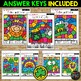# Color by Code St. Patrick's Day Math Activities {Addition & Subtraction to 20}Subject
Resource Type
File Type

PDF

Product Rating
Standards
Also included in:
1. Are you looking for fun and engaging things for your students to do around a holiday? Well, you have found it! Save money with this Color by Code Holiday Set! These are great for morning work, for early finishers, to use in math stations, and perfect for when you have a substitute! This set includes
\$36.00
\$26.50
Save \$9.50
• Product Description
• StandardsNEW

This is a fabulous set of holiday worksheets for your students! They feature St. Patrick's Day addition and subtraction color by code activities to twenty. There are eight different images available in full page and half page sizes! These are great for morning work, independent math stations, for early finishers, and when you have a substitute!

▶️Save \$\$\$\$\$\$\$\$\$\$\$ and grab this as part of my Color By Code Holiday Bundle to 20.▶️

This KID FRIENDLY set features 8 no prep worksheets:
Leprechaun Shoe and Flag Addition to 20

Leprechaun Subtraction to 20

Pot of Gold with a Rainbow Subtraction within 20

Pot of Gold with a Coin and Flag Addition and Subtraction to 20

Horse Shoe and Shamrock Addition and Subtraction to 20

Leprechaun Hat Addition and Subtraction to 20

All of these pages are included in a 1/2 sheet size option to save paper!

There are also answer key pages at the end! (Available in 1/2 sheets also)

You may also be interested in:
❤️ ST. PATRICK'S DAY BINGO {25 Different Bingo Cards}

❤️ St. Patrick's Day Bingo Game DIY {DO IT YOURSELF}

❤️ Word Problem Matching Game for St. Patrick's Day, First Grade

❤️ SAINT PATRICK'S DAY Activities Packet NO PREP Fun March

❤️ Subtraction with Regrouping Game, Spring Math Scoot {Self Check with QR Codes}

❤️ Color by Code Spring Math Activities {Addition and Subtraction to 10}

Remember, if you are not following me yet on TPT, be sure and do so!

Alisha
Busy Me Plus Three

Search Key Terms:
St. | Patrick | Patrick's | Saint | March | 17 | 17th | spring | holiday | holidays | code | color by code | color by number | number | addition | subtraction | mixed | number sentences | numbers | color | packet | mystery | picture | centers | Fun | Morning Work | stations | independent | centers | gift | mystery picture | kindergarten | first grade | second grade | math | half | page | full | activity| activities |

Fluently add and subtract within 20 using mental strategies. By end of Grade 2, know from memory all sums of two one-digit numbers.
Add and subtract within 20, demonstrating fluency for addition and subtraction within 10. Use strategies such as counting on; making ten (e.g., 8 + 6 = 8 + 2 + 4 = 10 + 4 = 14); decomposing a number leading to a ten (e.g., 13 - 4 = 13 - 3 - 1 = 10 - 1 = 9); using the relationship between addition and subtraction (e.g., knowing that 8 + 4 = 12, one knows 12 - 8 = 4); and creating equivalent but easier or known sums (e.g., adding 6 + 7 by creating the known equivalent 6 + 6 + 1 = 12 + 1 = 13).
Apply properties of operations as strategies to add and subtract. If 8 + 3 = 11 is known, then 3 + 8 = 11 is also known. (Commutative property of addition.) To add 2 + 6 + 4, the second two numbers can be added to make a ten, so 2 + 6 + 4 = 2 + 10 = 12. (Associative property of addition.)
Decompose numbers less than or equal to 10 into pairs in more than one way, e.g., by using objects or drawings, and record each decomposition by a drawing or equation (e.g., 5 = 2 + 3 and 5 = 4 + 1).
Solve addition and subtraction word problems, and add and subtract within 10, e.g., by using objects or drawings to represent the problem.
Total Pages
Included
Teaching Duration
N/A
Report this Resource to TpT
Reported resources will be reviewed by our team. Report this resource to let us know if this resource violates TpT’s content guidelines.